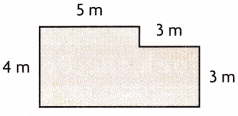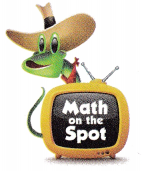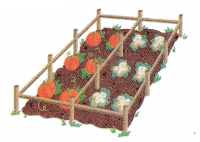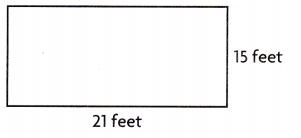# Texas Go Math Grade 5 Lesson 9.1 Answer Key Formulas for Area and Perimeter

Refer to our Texas Go Math Grade 5 Answer Key Pdf to score good marks in the exams.
Test yourself by practicing the problems from Texas Go Math Grade 5 Lesson 9.1
Answer Key Formulas for Area and Perimeter.

## Texas Go Math Grade 5 Lesson 9.1 Answer Key Formulas for Area and Perimeter

Unlock the Problem

A formula is an equation that expresses a mathematical rule.
You can use formulas to find the perimeter and area of rectangles.

Lloyd is planting a rectangular garden that measures 40 feet by 24 feet.
He wants to put a fence around it to protect his vegetables from rabbits.
How many feet of fencing does he need?

Use a formula to find the perimeter.
P = l + w + l + w, P = perimeter; l = length; w = width
P = 40 + ___24____ + __40_____ + ___24____ Replace the
unknowns with the lengths and the widths.
The perimeter is ___128____ feet. So, Lloyd needs ___128____ feet of fencing.

Remember
Area is measured in square units, such as square feet or sq ft.
The perimeter is 128 feet, So Lloyd needs 128 feet of fencing,

Explanation:
Lloyd is planting a rectangular garden that measures 40 feet by 24 feet.
He wants to put a fence around it to protect his vegetables from rabbits.
So number of feet of fencing does he need is using a formula to
find the perimeter P = l + w + l + w, P = perimeter; l = length; w = width is
P= 40 + 24 + 40 + 24 = 128 feet, So Lloyd needs 128 feet of fencing.

Lloyd needs to find how large his garden is so he can order enough mulch for the garden.
What is the area of Lloyd’s garden?

Use a formula to find the area.
A = l × w, A = area; I = length; w = width
A = ____40____ × __24_____ Replace the unknowns with the length and the width.
A = ____960_______ Multiply.
So, the area of Lloyd’s garden is _____960___ square feet.
The area of the garden is 960 square feet,

Explanation:
Given Lloyd is planting a rectangular garden that measures
length 40 feet by width 24 feet, therefore the area of
Lloyd’s garden is 40 X 24 = 960 square feet.

Try This!

You can also use the formula P = 2l + 2w to find the perimeter.
What is the perimeter of a rectangle that is 12 feet long and 16 feet wide?
P = 2 × ____12_____ + 2 × ___16_______
Replace the unknowns with the length and the width.
P = __24 + 32_______
The perimeter is ___56___ feet.
The perimeter is 56 feet,

Explanation:
Given to find the perimeter of a rectangle that is 12 feet long and 16 feet wide,
by using the formula p = 2l + 2w,
so p = 2 X 12 + 2 X 16,
p = 24 + 32,
p = 56 feet. therefore the perimeter is 56 feet.

Math Talk
Mathematical Processes

Explain how you can use the properties of operations to write P = l + w + l + w as P = 2l + 2w.
By using properties of operations addition we write
p = l + w + l + w as P = 2l + 2w,

Explanation:
Given to write p = l + w + l + w by using properties of operations addition
we add common terms l with l and w with w we get p = (l + l) + (w + w),
p = 2l + 2 w.

Example

Find the area.STEP 1: Separate the figure into a rectangle and a square.

STEP 2: Find the area of the rectangle.
A = l × w
A = __3 X 3_________
A = ___9________
The area of the rectangle is _____9______ square meters.

STEP 3: Find the area of the square.
A = ___5 X 4________
A = ____20_______
A = ___________
The area of the square is _____20______ square meters.

STEP 4: Find the area of the complex figure by adding the areas.
A = ____9_______ + ___20________
A = ____29_______
So, the area of the complex figure is ____29_______ square meters.
The area of the complex figure is 29 square meters,

Explanation:
STEP 1: Separate the figure into a rectangle and a square,
STEP 2: Finding the area of the rectangle as
A = l × w,
A = 3 X 3,
A = 9,
The area of the rectangle is 9 square meters,
STEP 3: Finding the area of the square
A = 5 X 4,
A = 20,
The area of the square is 20 square meters.

STEP 4: Find the area of the complex figure by adding the areas.
A = 9 + 20,
A = 29,
So, the area of the complex figure is 29 square meters.

Share and Show

Question 1.
Find the perimeter of the square.P = ____14_____ + ___14_____ + ___14______ + ___14______
P = _____56______
The perimeter is _____56______ meters
The perimeter of the square is 56 meters,

Explanation:
Given the side of the square is 14 meters,
therefore the perimeter of square is 14 + 14 + 14 + 14 = 56 meters.

Question 2.
Find the area of the rectangle.A = ____25___ × ____12_____
A = ____300_____
The area is ___300______ square feet.
Given width 25 feet and 12 feet wide rectangle,
Therefore area of rectangle is 25 X 12 = 300 square feet.

Problem Solving

Question 3.
H.O.T. Explain how you can use s to write the formula for the
perimeter of a square with side length s.
Perimeter= 4s,

Explanation:
Given s to write the formula for the perimeter of a square with side length s
is s + s + s + s =  4s.

Question 4.
H.O.T. A rectangle has an area of 96 square feet.
If the length of the rectangle is 12 feet,
what is the width of the rectangle?
8 feet is the width of the rectangle,

Explanation:
Given a rectangle has an area of 96 square feet.
If the length l of the rectangle is 12 feet, let w be the
width of the rectangle as we know area of rectangle
is A = l X w substituting 96 square feet= 12 feet X w,
w = 96 square feet  ÷ 12 feet = 8 feet.

Problem Solving

Question 5.
Brent plans to stain a deck that is 14 feet by 8 feet. If one can of
stain covers an area of 100 square feet, how many cans of stain
will he need? Explain.
2 cans of stain Brent need,

Explanation:
Brent plans to stain a deck that is 14 feet by 8 feet. If one can of
stain covers an area of 100 square feet, So a deck is of area
14 feet X 8 feet = 112 square feet  as one can of
stain covers an area of 100 square feet therefore  number of
cans of stain Brent will need is 2.

Question 6.
H.O.T. Multi-Step Latoya uses 50 feet of wood to make a rectangular garden bed.
If the length of the garden bed is 10 feet, what is the width?Width is 15 feet,

Explanation:
Latoya uses 50 feet of wood to make a rectangular garden bed,
So number of feet of fencing does he need is using a formula
for finding the perimeter P = l + w + l + w, P = perimeter; l = length; w = width is
50 = 10 + w + 10 + w upon solving we get 2w = 50 – 20 = 30,
2 w = 30 therefore w = 30 ÷ 2 = 15 feet.

Question 7.
H.O.T. What’s the Error? Maggie wants to fence off two side-by-side sections of her garden.
Each section is 14 feet long and 6 feet wide. She says she needs 80 feet of fencing.
Explain what is wrong with her thinking. How much fencing does she really need?Maggie really needs 160 feet of fencing not 80 feet,

Explanation:
Given Maggie wants to fence off two side-by-side sections of her garden
and each section is 14 feet long and 6 feet wide,
She says she needs 80 feet of fencing, but wrong with her thinking,
as if we see 80 feet(14 + 6 + 14 + 6) will cover only one section off the garden
fence for two side-by-side sections of her garden she needs 2 X 80 feet = 160 feet.

Fill in the bubble for the correct answer choice.

Question 8.
Apply Tina is fixing a rectangular sign. She plans to place metal trim around the sign edges.
The rectangle measures 32 inches by 9 inches. How much trim will Tina need?
(A) 36 inches
(B) 41 inches
(C) 72 inches
(D) 82 inches
(D) 82 inches,

Explanation:
Given Tina is fixing a rectangular sign. She plans to place
metal trim around the sign edges.
The rectangle measures 32 inches by 9 inches. So trim will Tina need is
32 inches + 9 inches + 32 inches + 9 inches = 82 inches which matches
with (D).

Question 9.
A rectangle has a length of 5 meters and a width of 4 meters.
Which equation can you use to find the perimeter?
(A) P = 4 × 5
(B) P = 4 × 4
(C) P = 4 + 4 + 5 + 5
(D) P = 4 + 5
(C) P = 4 + 4 + 5 + 5,

Explanation:
Given rectangle has a length of 5 meters and a width of 4 meters
we know perimeter P = l + w + l + w, where P = perimeter; l = length;
w = width so we get the equation to find the perimeter is
P = 4 + 4 + 5 + 5 which matches with (C).

Question 10.
Multi-Step Lana had an “L” shaped piece of felt. Her mom cut it into two rectangles.
One rectangle measured 4 inches by 9 inches, and the other measured 4 inches by 3 inches.
What is the total area of the two rectangles?
(A) 40 square inches
(B) 48 square inches
(C) 72 square inches
(D) 24 square inches
(B) 48 square inches,

Explanation:
Given Lana had an “L” shaped piece of felt. Her mom cut it into two rectangles.
One rectangle measured 4 inches by 9 inches, and the other measured 4 inches by 3 inches.
So area of one rectangle is 4 inches X 9 inches = 36 square inches,
other area of rectangle is 4 inches X 3 inches = 12 square inches,
so the total area of the two rectangles is
36 square inches + 12 square inches = 48 square inches which matches
with (B).

TEXAS Test Prep

Question 11.
Mai wants to tile the floor of her kitchen.
Each tile has an area of 1 square foot. The floor of her kitchen is
11 feet by 16 feet. How many tiles does she need?
(A) 150
(B) 54
(C) 176
(D) 352
(C) 176,

Explanation:
Given Mai wants to tile the floor of her kitchen.
Each tile has an area of 1 square foot. The floor of her kitchen is
11 feet by 16 feet. So total area of Mai kitchen is
11 feet X 16 feet = 176 square foot matches with (c).

### Texas Go Math Grade 5 Lesson 9.1 Homework and Practice Answer Key

Question 1.
Find the perimeter of the rectangle.P = ___21__ + ____15___ + ___21___ + ___15____
P = _____72_______
The perimeter is _____72_______ feet.
The perimeter of rectangle is 72 feet,

Explanation:
Given rectangle has a length of 21 feet and a width of 15 feet
we know perimeter P = l + w + l + w, where P = perimeter; l = length;
w = width so the perimeter of rectangle is P = 21 + 15 + 21 + 15 = 72 feet.

Question 2.
Find the area of the square.A = ___17______ × ___17_______
A = ___289_____
The area is ____289___ square inches.
The area of the given square is 289 square inches,

Explanation:
Given the side of the square is 17 inches,
the area of square is 17 inches X 17 inches = 289 square inches.

Question 3.
A rectangle has a perimeter of 68 inches. 1f the width of the rectangle is 10 inches,
what is the length of the rectangle? Explain how you know.
The length of the rectangle is 24 inches,

Explanation:
Given a rectangle that has a perimeter of 68  inches.
If the width of the rectangle is 10 inches, let l be the
length of the rectangle as we know perimeter P = l + w + l + w,
where P = perimeter; l = length; w = width so the length of rectangle l is
68 = 10 + 10 + l + l, so 2l = 68 – 20 = 48, l = 48 ÷ 2 = 24 inches.

Question 4.
A square has an area of 81 square feet. What is the length of
each side of the square? Explain how you know.
The length of side of the square is 9 feet,

Explanation:
Given a square has an area of 81 square feet. The length of
each side of the square will be as area of square is s X s ,
so 81 square feet = s X s,
s X s = 9 feet X 9 feet , therefore s = 9 feet.

Problem Solving

Question 5.
Lea wants to put a fence around her garden. Her garden measures 14 feet by 15 feet.
She has 50 feet of fencing. How many more feet of fencing does
Lea need to put a fence around her garden?

Question 6.
Lea wants to put a new layer of soil on her 14 feet by 15 feet garden.
She finds the area of her garden so she knows how much soil to buy.
If one bag of soil covers 20 square feet, how many bags of soil will Lea need? Explain.
11 bags of soil Lea needs,

Explanation:
Given Lea wants to put a new layer of soil on her 14 feet by 15 feet garden.
She finds the area of her garden as 14 feet X 15 feet = 210 square feet,
she knows how much soil to buy If one bag of soil covers 20 square feet,
210 square feet requires 210 ÷ 20 = 10 bags remainder 10 square feet,
therefore 11 bags of soil Lea needs.

Lesson Check

Question 7.
A soccer field has a length of 100 yards and a width of 60 yards.
Which equation can you use to find the area of the soccer field?
(A) A = 100 × 60
(B) A = 100 + 60 + 100 + 60
(C) A = 100 + 60
(D) A = 160 × 4
(A) A = 100 × 60,

Explanation:
Given a soccer field has a length of 100 yards and a width of 60 yards,
as we know area of square is Area is equal to length X width
so the equation for the area of the soccer field is A = 100 X 60 which
matches with (A).

Question 8.
A baseball diamond is a square with a perimeter of 360 feet.
What is the length of one side?
(A) 80 feet
(B) 180 feet
(C) 90 feet
(D) 60 feet
(C) 90 feet,

Explanation:
Given a baseball diamond is a square with a perimeter of 360 feet.
So the length of one side will be as perimeter of square with side s is
p = 4s so 360 feet = 4 X s, therefore one side is 360 ÷ 4 = 90 feet matches
with (C).

Question 9.
Zoey wants to cover her bedroom floor with carpet squares.
Each square has an area of 1 square foot.
Her bedroom measures 12 feet by 14 feet.
How many carpet squares does Zoey need?
(A) 168
(B) 144
(C) 336
(D) 52
(A) 168,

Explanation:
Given Zoey wants to cover her bedroom floor with carpet squares.
Each square has an area of 1 square foot.
Her bedroom measures 12 feet by 14 feet.
So number of squares does Zoey need is 12 X 14 = 168 square feet
matches with (A).

Question 10.
Edward wants to put a string of lights around a rectangular window
that is 32 inches wide and 40 inches high. How long will the string of lights
need to be to go around the window?
(A) 72 inches
(B) 1,280 inches
(C) 144 inches
(D) 112 inches
(B) 1,280 inches,

Explanation:
Given Edward wants to put a string of lights around a rectangular window
that is 32 inches wide and 40 inches high. The string of lights
need to be to go around the window is 32 X 40 = 1,280 inches matches
with (B) 1,280 inches.

Question 11.
Multi-Step Chantal buys two small rugs for her kitchen.
One rug measures 3 feet by 5 feet. The other rug measures 4 feet by 6 feet.
What is the area of the part of the kitchen the two rugs will cover?
(A) 39 square feet
(B) 30 square feet
(C) 24 square feet
(D) 36 square feet
(A) 39 square feet,

Explanation:
Given Chantal buys two small rugs for her kitchen.
One rug measures 3 feet by 5 feet. The other rug measures 4 feet by 6 feet.
First rug covers 3 feet by 5feet is 5 X 3 = 15 square feet,
Other rug covers 4 feet by 6 feet is 4 X 6 = 24 square feet,
The area of the part of the kitchen the two rugs will cover is
15 square feet + 24 square feet = 39 square feet.

Question 12.
Multi-Step Isaac is painting a wall that is 9 feet by 18 feet. So far,
he has painted a part of the wall that is a 4 feet by 7 feet rectangle.
What is the area of the part of the wall that Isaac has left to paint?
(A) 190 square feet
(B) 134 square feet
(C) 22 square feet
(D) 151 square feet